### MEMO 2017 ekipno problem 4

Kvaliteta:
Avg: 0,0
Težina:
Avg: 6,0

Let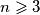$n \geqslant 3$ be an integer. A sequence$P_1, P_2, \ldots, P_n$ of distinct points in the plane is called good if no three of them are collinear, the polyline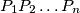$P_1P_2 \ldots P_n$ is non-self-intersecting and the triangle$P_iP_{i + 1}P_{i + 2}$ is oriented counterclockwise for every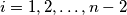$i = 1, 2, \ldots, n - 2$. For every integer$n \geqslant 3$ determine the greatest possible integer$k$ with the following property: there exist$n$ distinct points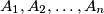$A_1, A_2, \ldots, A_n$ in the plane for which there are$k$ distinct permutations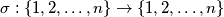$\sigma : \{1, 2, \ldots, n\} \to \{1, 2, \ldots, n\}$ such that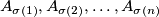$A_{\sigma(1)}, A_{\sigma(2)}, \ldots, A_{\sigma(n)}$ is good.
(A polyline$P_1P_2 \ldots P_n$ consists of the segments$P_1P_2, P_2P_3, \ldots, P_{n - 1}P_n$.)

Izvor: Srednjoeuropska matematička olimpijada 2017, ekipno natjecanje, problem 4